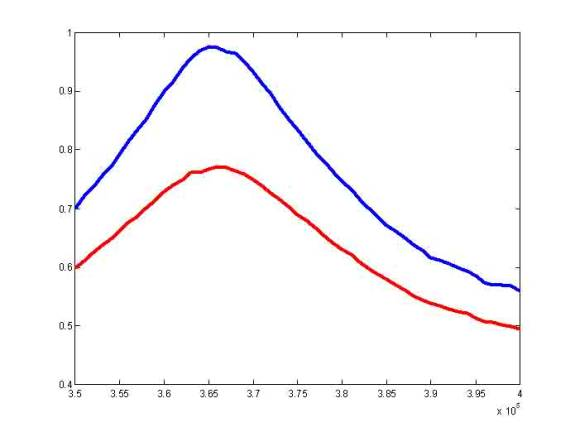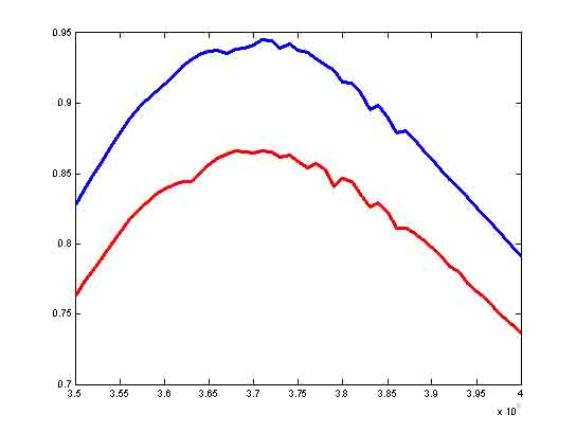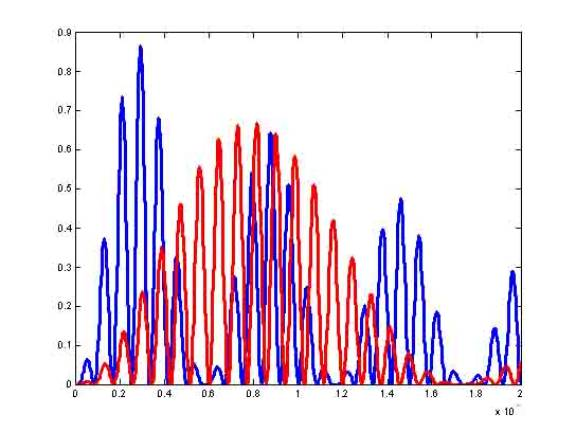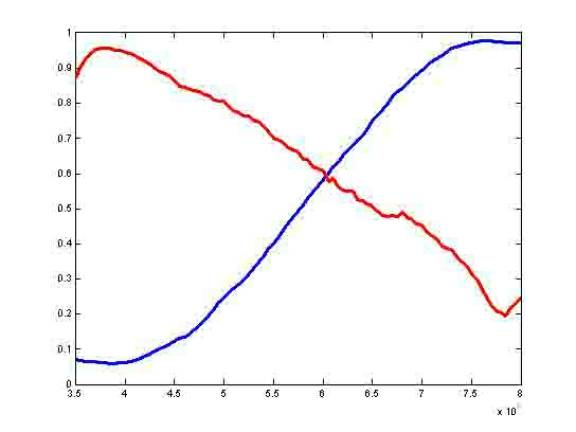Matlab analysis of OLTCs DRSSTCs and untuned primary SSTCsThis plot shows the efficiency of my DRSSTC, at a coupling of 0.1 with and without the secondary loss. I think you can figure out which one is which ;^). The x axis is the drive frequency, in rad/sec with the resonant frequency held constant at 375K.This one is the same thing, except the k is 0.28This plot shows efficiency on the y axis, and time on the x axis for an OLTC without secondary loss. Blue is k=0.28, red is k=0.1. The reason the plot is not smooth is that efficiency is defined as energy in c2/initial energy in c1.This is for a SSTC with an untuned primary. The k is 0.35 and the resonant frequency is the same for the red line, and doubled for the blue one. The higher frequency works better, due to less magnifying current. The peak Efficiency is at the poles not the zero, even though the secondary's capacitive current partially cancels magnetizing current. Because of the hard switching at high current, the efficiency might go down 1/2 or 1%, but the effect is negligible.

The secondary inductor and toroid are the same for all three coils, (with the exception of the blue line on the last one, because the secondary inductance is down by a factor of 4) and the energy transfer is also the same. The amount of IGBTs is also the same, so the OLTC and DRSSTC with k of 0.28 should be a little more efficient, but it would cost more IGBTs. The drive voltage for the DRSSTC is 400 volts, and the primary voltage for the OLTC is 700 volts.

There is also the cost of capacitors. DRSSTCs only need a quarter of the amount of capacitors that the OLTC needs, but the untuned primary SSTCs don't need any. As far as cost goes, untuned primary SSTCs might do best, but they would be slightly less efficient, and designing it might be tough because of hard switching at high current, and the huge current offset.

Here are the Matlab files